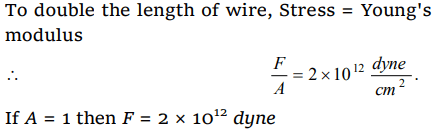## Mechanical Properties of Solids Questions and Answers Part-1

1.The ratio of the lengths of two wires A and B of same material is 1 : 2 and the ratio of their diameter is 2 : 1. They are stretched by the same force, then the ratio of increase in length will be
a) 2 : 1
b) 1 : 4
c) 1 : 8
d) 8 : 1

Explanation: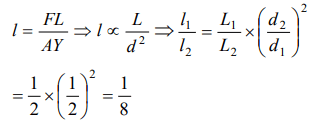2. The Young's modulus of a wire of length L and radius r is Y $N/m^{2}$. If the length and radius are reduced to L/2 and r/2, then its Young's modulus will be
a) Y/2
b) Y
c) 2Y
d) 4Y

Explanation: Young's modulus of wire does not varies with dimension of wire. It is the property of given material.

3. A beam of metal supported at the two ends is loaded at the centre. The depression at the centre is proportional to
a) $Y^{2}$
b) Y
c) 1/Y
d) $1\diagup Y^{2}$

Explanation: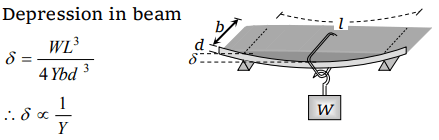4. When a certain weight is suspended from a long uniform wire, its length increases by one cm. If the same weight is suspended from another wire of the same material and length but having a diameter half of the first one then the increase in length will be
a) 0.5 cm
b) 2 cm
c) 4 cm
d) 8 cm

Explanation: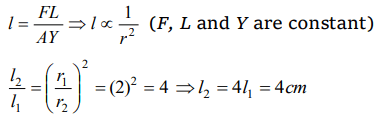5. Hook's law defines
a) Stress
b) Strain
c) Modulus of elasticity
d) Elastic limit

Explanation: Modulus of elasticity

6.A wire is loaded by 6 kg at its one end, the increase in length is 12 mm. If the radius of thewire is doubled and all other magnitudes are unchanged, then increase in length will be
a) 6 mm
b) 3 mm
c) 24 mm
d) 48 mm

Explanation:7. The area of cross-section of a wire of length 1.1 metre is 1 mm2. It is loaded with 1 kg. If Young's modulus of copper is $1.1\times10^{11}N\diagup m^{2}$  , then the increase in length will be if $\left(g=10m\diagup s^{2}\right)$
a) 0.01 mm
b) 0.075 mm
c) 0.1 mm
d) 0. 15 mm

Explanation:8. On increasing the length by 0.5 mm in a steel wire of length 2 m and area of cross-section 2 mm2 , the force required is [Y for steel $=2.2\times10^{11}N\diagup m^{2}$     ]
a) $1.1\times10^{5}N$
b) $1.1\times10^{4}N$
c) $1.1\times10^{3}N$
d) $1.1\times10^{2}N$

Explanation: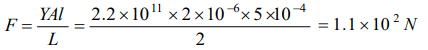9. If Young's modulus of iron is $2\times10^{11}N\diagup m^{2}$   and the interatomic spacing between two molecules is $3\times10^{-10}$   metre , the interatomic force constant is
a) 60 N/m
b) 120 N/m
c) 30 N/m
d) 180 N/m10.In CGS system, the Young's modulus of a steel wire is $2\times10^{12}$ . To double the length of a wire of unit cross-section area, the force required is
a) $4\times10^{6}dynes$
b) $2\times10^{12}dynes$
c) $2\times10^{12}newtons$
d) $2\times10^{8}dynes$